Courses

# Formula Sheet: Thermodynamics Class 12 Notes | EduRev

## JEE : Formula Sheet: Thermodynamics Class 12 Notes | EduRev

The document Formula Sheet: Thermodynamics Class 12 Notes | EduRev is a part of the JEE Course Chemistry Class 12.
All you need of JEE at this link: JEE

Thermodynamic processes :

• Isothermal process: T = constant
dT = 0
ΔT = 0
• Isochoric process: V = constant
dV = 0
ΔV = 0
• Isobaric process: P = constant
dP = 0
ΔP = 0
• Adiabatic process: q = 0
or heat exchange with the surrounding = O(zero)

IUPAC Sign convention about Heat and Work :
Work done on the system = Positive
Work done by the system = Negative

1st Law of Thermodynamics
ΔU = (U- U1) = q + w

Law of equipartion of energy :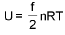(only for ideal gas)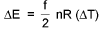where f= degrees of freedom for that gas. (Translational + Rotational)
f = 3 formonoatomic
= 5 for diatomic or linear polyatmic
= 6 for non - linear polyatmic

Calculation of heat (q):
Total heat capacity: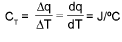Molar heat capacity :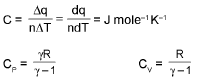Specific heat capacity (s):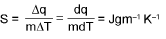WORK DONE (w) :
Isothermal Reversible expansion/compression of an ideal gas :

W = - nRT In (Vf/Vi)

Reversible and irreversible isochoric processes.
Since dV = 0
So dW = - Pext . dV = 0.

Reversible isobaric process:
W = P (Vf - Vi)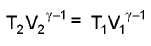Reversible Work: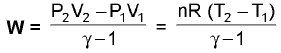Irreversible Work :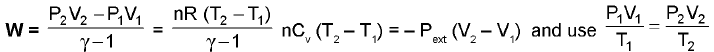Free expansion - Always going to be irrerversible and since Pext = 0
so dW = -Pext..dV = 0
If no. heat is supplied q = 0
then ΔE = 0 so ΔT = 0.

Application of 1st Law :
ΔU = ΔQ + ΔW ⇒ ΔW = -P ΔV
∴ ΔU = ΔQ -PΔV

Constant volume process
Heat given at constant volume = change in internal energy
∴du = (dq)v
du = nCvdT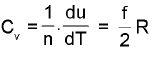Constant pressure process:
H ≡ Enthalpy (state function and extensive property)
H = U + PV

⇒ Cp - Cv = R (only for ideal gas)

Second Law Of Thermodynamics :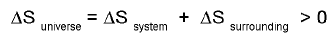fo r a spontaneous process.

Entropy (S):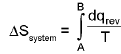Entropy calculation for an ideal gas undergoin a process :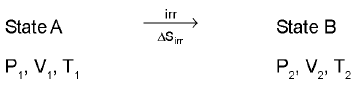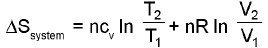(only for an ideal gas)

Third Law Of Thermodynamics :
The entropy of perfect crystals of all pure elements & compounds is zero at the absolute zero of temperature.

Gibb’s free energy (G) : (State function and an extensive property)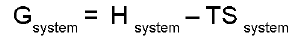Criteria of spontaneity:
(i) If ΔGsystem is (-ve) < 0 ⇒ process is spontaneous
(ii) If ΔGsystem is > 0 ⇒ process is non spontaneous
(iii) lf ΔGsystem  = 0 = 3 system is at equilibrium.

Physical interpretation of ΔG :
The maximum amount of non-expansional (compression) work which can be performed.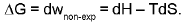Standard Free Energy Change (ΔG°) :
1. ΔG° = -2.303 RTIog10K
2. At equilibrium ΔG = 0.
3. The decrease in free energy (-ΔG) is given as :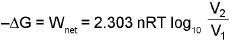4.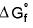for elemental state = 0

5.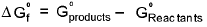Thermochemistry:
Change in standard enthalpy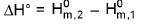= heat added at constant pressure. =  CPΔT.
If Hproducts > Hreactants

• Reaction should be end other micas we have to give extra heat to reactants to get these converted into products and if
Hproducts > Hreactants
• Reaction will be exothermic as extra heat content of reactants will be released during the reaction. Enthalpy change of a reaction :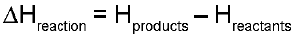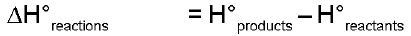= positive - endothermic
= negative - exothermic

Temperature Dependence Of ΔH : (Kirchoff's equation):
For a constant volume reaction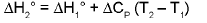where ΔCP = Cp (products) - Cp (reactants).
For a constant volume reaction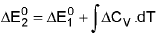Enthalpy of Reaction from Enthalpies of Formation :
The enthalpy of reaction can be calculated by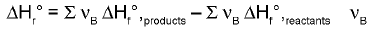is the stoichiometric coefficient.

Estimation of Enthalpy of a reaction from bond Enthalpies :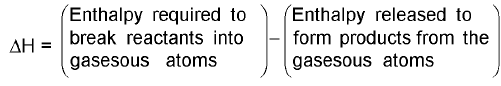Resonance Energy: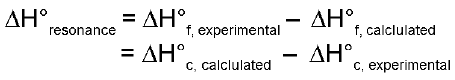CHEMICAL EQUILIBRIUM
At equilibrium :
(i) Rate of forward reaction = rate of backward reaction

(ii) Concentration (mole/litre) of reactant and product becomes constant.

(iii) ΔG = 0.
(iv) Q = Keq

Equilibrium constant (K) :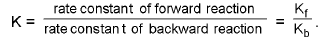Equilibrium constant in terms of concentration (Kc) :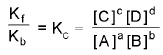Equilibrium constant in terms of partial pressure (Kp):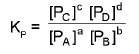Equilibrium constant in terms of mole fraction (Kx) :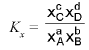Relation between Kp & Kc :
Kp = Kc.(RT)Δn.

Relation between KP & KX :
KP = KX(P)Δn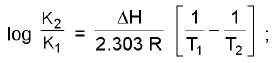ΔH = Enthalpy of reaction

Relation between equilibrium constant & standard free energy change :
ΔG° = - 2.303 RT log K

Reaction Quotient (Q):
The values of expression Q =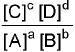Degree of Dissociation (α):
α = no. of moles dissociated / initial no. of moles taken
= fraction of moles dissociated out of 1 mole.
Note: % dissociation = α x 100
Observed molecular weight and Observed Vapour Density of the mixture :
Observed molecular weight of An(g) =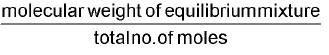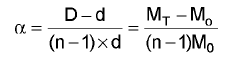External factor affecting equilibrium :
Le Chatelier's Principle:
If a system at equilibrium is subjected to a disturbance or stress that changes any of the factors that determine the state of equilibrium, the system will react in such a way as to minimize the effect of the disturbance.

Effect of concentration:

• If the concentration of reactant is increased at equilibrium then reaction shift in the forward direction .
• If the concentration of product is increased then equilibrium shifts in the backward direction

Effect of volume:

• If volume is increased pressure decreases hence reaction will shift in the direction in which pressure increases that is in the direction in which number of moles of gases increases and vice versa.
• If volume is increased then, for
Δn > 0 reaction will shift in the forward direction
Δn < 0 reaction will shift in the backward direction
Δn = 0 reaction will not shift.

Effect of pressure:
If pressure is increased at equilibrium then reaction will try to decrease the pressure, hence it will shift in the direction in which less no. of moles of gases are formed.

Effect of inert gas addition :
(i) Constant pressure: If inert gas is added then to maintain the pressure constant, volume is increased. Hence equilibrium will shift in the direction in which larger no. of moles of gas is formed
Δn > 0 reaction will shift in the forward direction
Δn < 0 reaction will shift in the backward direction
Δn = 0 reaction will not shift.
(ii) Constant volume: Inert gas addition has no effect at constant volume.

Effect of Temperature:
Equilibrium constant is only dependent upon the temperature.
If plot of lnk vs 1/T is plotted then it is a straight line with slope =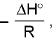and intercept =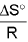• For endothermic (ΔH > 0) reaction value of the equilibrium constant increases with the rise in temperature
• For exothermic (ΔH < 0) reaction, value of the equilibrium constant decreases with increase in temperature
• For ΔH > 0, reaction shifts in the forward direction with increase in temperatutre
• For ΔH < 0, reaction shifts in the backward direction with increases in temperature.
• If the concentration of reactant is increased at equilibrium then reaction shift in the forward direction .
• If the concentration of product is increased then equilibrium shifts in the backward direction

Vapour Pressure of Liquid
Relative Humidity =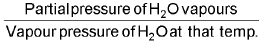Thermodynamics of Equilibrium :
ΔG = ΔG°+ 2.303 RT log10Q
Vant Hoff equation-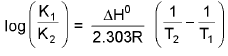IONIC EQUILIBRIUM
OSTWALD DILUTION LAW:

• Dissociation constant of weak acid (Ka),If α « 1 , then 1 - α ≌ 1 or Ka = cα2 or α =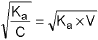• Similarly for a weak base,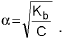Higher the value of Ka/ Kb, strong is the acid/base.

Acidity and pH scale :
∴ pH = - log aH+  (where aH+ is the activity of H+ ions = molar concentration for dilute solution).
[Note : pH can also be negative or > 14]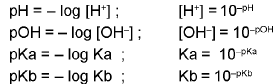PROPERTIES OF WATER :

1. In pure water [H+] = [OH-] so it is Neutral.
2. Moler concentration / Molarity of water = 55.56 M.
3. Ionic product of water (Kw) :
Kw= [H+][OH-] = 10-14 at 25° (experimentally)
pH = 7 = pOH ⇒ neutral
pH < 7 or pOH >7 ⇒ acidic
pH>7or pOH<7 ⇒ Basic
4. Degree of dissociation of water: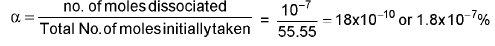5. Absolute dissociation constant of water :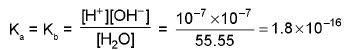pK= pKb = - log (1.8 x 10-16) = 16 - log 1.8 = 15.74
Ka x Kb = [H+] [OH-] = Kw
⇒ Note: for a conjugate acid- base pairs
pKa + pKb = pKw = 14 at 25°C.
pKa of H3O+ ions = -1.74
pKb of OH- ions = -1.74.

pH Calculations of Different Types of Solutions :

• Strong acid solution :
(i) If concentration is greater than 10-6M
In this case H+ ions coming from water can be neglected,
(ii) If concentration is less than 10-6 M
In this case H+ ions coming from water cannot be neglected
• Strong base solution :
Using similar method as in part (a) calculate first [OH-] and then use [H+] x [OH-] = 10-14
• pH of mixture of two strong acids :
Number of H+ ions from I-solution = N1 V1
Number of H+ ions from II-solution = N2 V2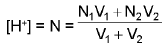• pH of mixture of two strong bases :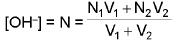• pH of mixture of a strong acid and a strong base :
If N1V1 > N2V2, then solution will be acidic in nature and [H+] = N =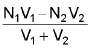If N2V2 > N1V1, then solution will be basic in nature and [OH-] = N =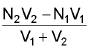• pH of a weak acid(monoprotic) solution :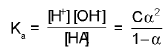if a << 1 ⇒ (1 - α) = 1 ⇒ Ka = Cα2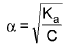(is valid if α < 0.1 or 10%)
On increasing the dilution ⇒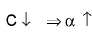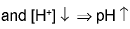(g) pH of a solution of a polyprotic weak acid :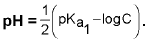RELATIVE STRENGTH OF TWO ACIDS :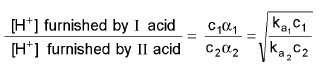• pH of a mixture of two weak acid(both monoprotic) solutions :
(α1« 1) and (α2 « 1) ⇒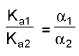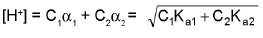If water is again considered third weak acid in solution of two weak acid then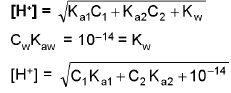• pH of a mixture of weak acid(monoprotic) and a strong acid solution :
If [SA] = C1 and [WA] =C2, then [H+] from SA = C1 and [H+] from WA = C2 Let HA is a weak acid.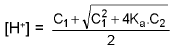If a strong acid of low cone is added in water then [H+] of solution can be calculated as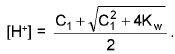SALT HYDROLYSIS :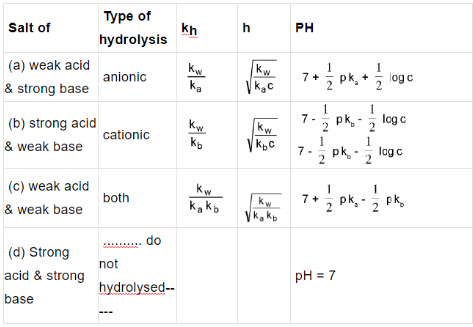Hydrolysis of ployvalent anions or cations
For [Na3PO4] = C.
Ka1 x Kh3 = Kw
Ka1 x Kh2 = Kw
Ka3 x Kh1 = Kw
Generally pH is calculated only using the first step Hydrolysis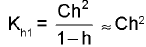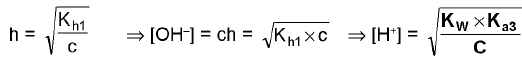So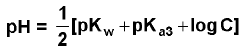Hydrolysis of Amphiprotic Anion. (Cation is not Hydrolysed e.g. NaHCO3, NaHS, etc.)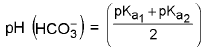Similarly for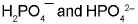amphiprotic anions.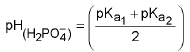and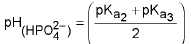•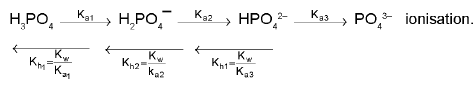The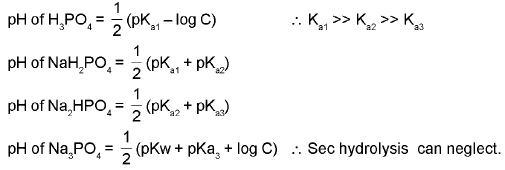BUFFER SOLUTION :
(a) Acidic Buffer: e.g. CH3COOH and CH3COONa. (weak acid and salt of its conjugate base).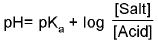[Henderson's equation]
(b) Basic Buffer: e.g. NH4OH + NH4CI. (weak base and salt of its conjugate acid).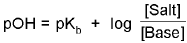Buffer capacity (index) :
Buffer capacity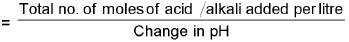Buffer capacity =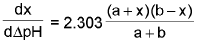INDICATOR :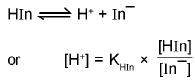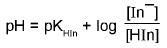⇒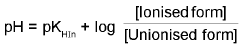SIGNIFICANCE OF INDICATORS :
Extent of reaction of different bases with acid (HCI) using two indicators :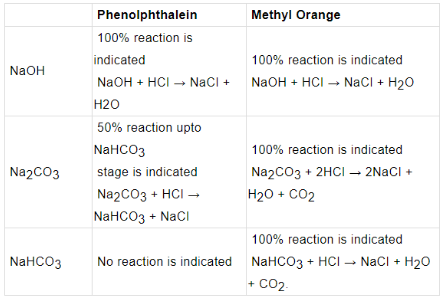ISOELECTRIC POINT: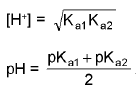SOLUBILITY PRODUCT :
Ksp = (xs)x (ys)y = xx.yy.(s)x+y

CONDITION FOR PRECIPITATION :
If ionic product KI.P > Ksp precipitation occurs,
if KI.P = Ksp saturated solution (precipitation just begins or is just prevented).

Offer running on EduRev: Apply code STAYHOME200 to get INR 200 off on our premium plan EduRev Infinity!

## Chemistry Class 12

106 videos|246 docs|198 tests

,

,

,

,

,

,

,

,

,

,

,

,

,

,

,

,

,

,

,

,

,

;